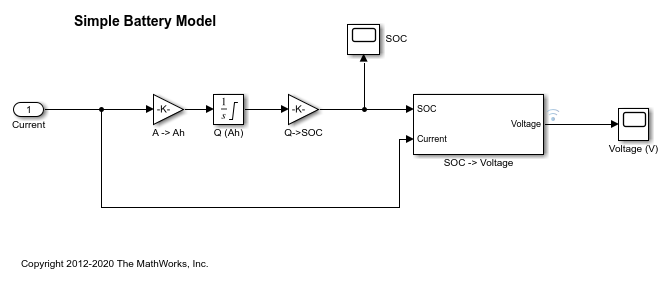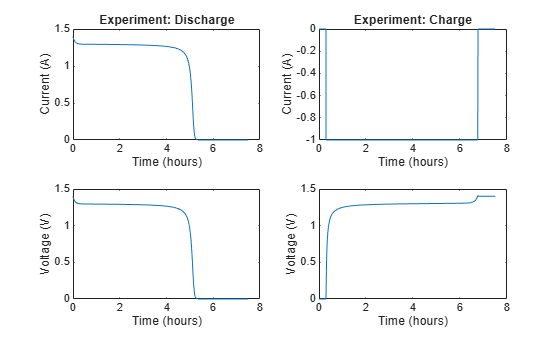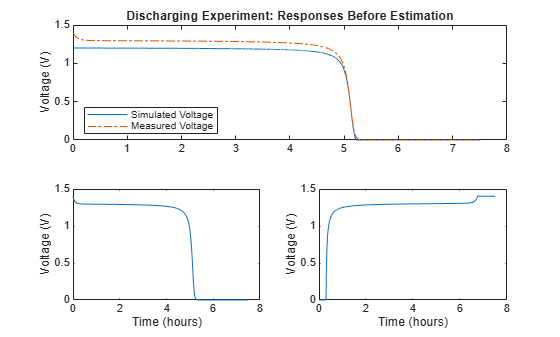# Estimate Model Parameters Per Experiment (Code)

This example shows how to use multiple experiments to estimate a mix of model parameter values; some that are estimated using all the experiments and others that are estimated using individual experiments. The example also shows how to configure estimation experiments with experiment dependent parameter values.

You estimate the parameters of a rechargeable battery based on data collected in experiments that discharge and charge the battery.

### Open the Model and Get Experimental Data

This example estimates parameters of a simple, rechargeable battery model, `sdoBattery`. The model input is the battery current and the model output, the battery terminal voltage, is computed from the battery state-of-charge.

`open_system('sdoBattery');`The model is based on the equation

`$\mathit{E}=\left(1-\mathrm{Loss}\right)\mathit{V}-\mathit{K}{\mathit{Q}}_{\mathrm{max}}\frac{1-\mathit{s}}{\mathit{s}}$`

In the equation:

$\mathit{E}$ is the battery terminal voltage in Volts.

$\mathit{V}$ is the battery constant voltage in Volts.

$\mathit{K}$ is the battery polarization resistance in Ohms.

${\mathit{Q}}_{\mathrm{max}}$ is the maximum battery capacity in Ampere-hours.

$\mathit{s}$ is the battery charge state, with 1 being fully charged and 0 discharged. The battery state-of-charge is computed from the integral of the battery current with a positive current indicating discharge and a negative current indicating charging. The battery initial state-of-charge is specified by ${\mathit{Q}}_{0}$ in Ampere-hours.

$\mathrm{Loss}$ is the voltage drop when charging, expressed as a fraction of the battery constant voltage. When the battery is discharging this value is zero.

`V`, `K`, `Qmax`, `Q0`, and `Loss` are variables defined in the model workspace.

Load the experiment data. A 1.2V (6500mAh) battery was subjected to a discharge experiment and a charging experiment.

`load sdoBattery_ExperimentData`

The variables `Charge_Data` and `DCharge_Data` are loaded into the workspace. The first column of `Charge_Data` contains time data. The second and third columns of `Charge_Data` describe the current and voltage during a battery charging experiment. `DCharge_Data` is similarly structured and contains data for a battery discharging experiment.

### Plot the Experiment Data

```subplot(221), plot(DCharge_Data(:,1)/3600,DCharge_Data(:,2)) title('Experiment: Discharge') xlabel('Time (hours)') ylabel('Current (A)') subplot(223) plot(DCharge_Data(:,1)/3600,DCharge_Data(:,3)) xlabel('Time (hours)') ylabel('Voltage (V)') subplot(222), plot(Charge_Data(:,1)/3600,Charge_Data(:,2)) title('Experiment: Charge') xlabel('Time (hours)') ylabel('Current (A)') subplot(224) plot(Charge_Data(:,1)/3600,Charge_Data(:,3)) xlabel('Time (hours)') ylabel('Voltage (V)')```### Define the Estimation Experiments

Create a 2-element array of experiment objects to specify the measured data for the two experiments.

Create an experiment object for the battery discharge experiment. The measured current data is specified as a timeseries in the experiment object.

`DCharge_Exp = sdo.Experiment('sdoBattery');`

Specify the input data (current) as a timeseries object.

`DCharge_Exp.InputData = timeseries(DCharge_Data(:,2),DCharge_Data(:,1));`

Create an object to specify the measured voltage output data.

```VoltageSig = Simulink.SimulationData.Signal; VoltageSig.Name = 'Voltage'; VoltageSig.BlockPath = 'sdoBattery/SOC -> Voltage'; VoltageSig.PortType = 'outport'; VoltageSig.PortIndex = 1; VoltageSig.Values = timeseries(DCharge_Data(:,3),DCharge_Data(:,1));```

Add the voltage signal to the discharge experiment as the expected output data.

`DCharge_Exp.OutputData = VoltageSig;`

Specify the battery initial charge state for the experiment. The battery charge state is modeled by the `Q (Ah)` block and it's initial value is specified by the variable `Q0`. Create a parameter for the `Q0` variable and add the parameter to the experiment. `Q0` is experiment dependent and assumes different values in the discharging and charging experiments.

```Q0 = sdo.getParameterFromModel('sdoBattery','Q0'); Q0.Value = 6.5; Q0.Free = false;```

`Q0.Free` is set to `false` because the initial battery charge is known and does not need to be estimated.

Add the `Q0` parameter to the experiment.

`DCharge_Exp.Parameters = Q0;`

Create an experiment object to store the charging experiment data. Add the measured current input and measured voltage output data to the object.

```Charge_Exp = sdo.Experiment('sdoBattery'); Charge_Exp.InputData = timeseries(Charge_Data(:,2),Charge_Data(:,1)); VoltageSig.Values = timeseries(Charge_Data(:,3),Charge_Data(:,1)); Charge_Exp.OutputData = VoltageSig;```

Add the battery initial charge and charging loss fraction parameters to the experiment. For this experiment, the initial charge (`Q0`) is known (0 Ah), but the value of the charging loss fraction (`Loss`) is not known.

```Q0.Value = 0; Loss = sdo.getParameterFromModel('sdoBattery','Loss'); Loss.Free = true; Loss.Minimum = 0; Loss.Maximum = 0.5; Charge_Exp.Parameters = [Q0;Loss];```

`Loss.Free` is set to true so that the value of `Loss` is estimated.

Collect both experiments into one vector.

`Exp = [DCharge_Exp; Charge_Exp];`

### Compare the Measured Output and the Initial Simulated Output

Create a simulation scenario using the first (discharging) experiment and obtain the simulated output.

```Simulator = createSimulator(Exp(1)); Simulator = sim(Simulator);```

Search for the voltage signal in the logged simulation data.

```SimLog = find(Simulator.LoggedData,get_param('sdoBattery','SignalLoggingName')); Voltage(1) = find(SimLog,'Voltage');```

Obtain the simulated voltage signal for the second (charging) experiment.

```Simulator = createSimulator(Exp(2),Simulator); Simulator = sim(Simulator); SimLog = find(Simulator.LoggedData,get_param('sdoBattery','SignalLoggingName')); Voltage(2) = find(SimLog,'Voltage');```

Plot the measured and simulated data. The model response does not match the experimental output data.

```subplot(211) plot(... Voltage(1).Values.Time/3600,Voltage(1).Values.Data, ... Exp(1).OutputData.Values.Time/3600, Exp(1).OutputData.Values.Data,'-.') title('Discharging Experiment: Simulated and Measured Responses Before Estimation') ylabel('Voltage (V)') legend('Simulated Voltage','Measured Voltage','Location','SouthWest')``````subplot(212) plot(... Voltage(2).Values.Time/3600,Voltage(2).Values.Data, ... Exp(2).OutputData.Values.Time/3600, Exp(2).OutputData.Values.Data,'-.') title('Charging Experiment: Simulated and Measured Responses Before Estimation') xlabel('Time (hours)') ylabel('Voltage (V)') legend('Simulated Voltage','Measured Voltage','Location','SouthEast') ```

### Specify Parameters to Estimate

Estimate the values of the battery voltage `V`, the battery polarization resistance `K`, and the charging loss fraction `Loss`. The `V` and `K` parameters are estimated using all the experiment data while the `Loss` parameter is estimated using only the charging data.

Select the battery voltage `V` and the battery polarization resistance `K` parameters from the model. Specify minimum and maximum bounds for these parameters.

```p = sdo.getParameterFromModel('sdoBattery',{'V','K'}); p(1).Minimum = 0; p(1).Maximum = 2; p(2).Minimum = 1e-6; p(2).Maximum = 1e-1;```

Get the experiment-specific `Loss` parameter from the experiment.

`s = getValuesToEstimate(Exp);`

Group all the parameters to be estimated.

`v = [p;s]`
``` v(1,1) = Name: 'V' Value: 1.2000 Minimum: 0 Maximum: 2 Free: 1 Scale: 2 Info: [1x1 struct] v(2,1) = Name: 'K' Value: 1.0000e-03 Minimum: 1.0000e-06 Maximum: 0.1000 Free: 1 Scale: 0.0020 Info: [1x1 struct] v(3,1) = Name: 'Loss' Value: 0.0100 Minimum: 0 Maximum: 0.5000 Free: 1 Scale: 0.0156 Info: [1x1 struct] 3x1 param.Continuous ```

### Define the Estimation Objective

Create an estimation objective function to evaluate how closely the simulation output, generated using the estimated parameter values, matches the measured data.

Use an anonymous function with one input argument that calls the `sdoBattery_Objective` function. We pass the anonymous function to `sdo.optimize`, which evaluates the function at each optimization iteration.

`estFcn = @(v) sdoBattery_Objective(v,Simulator,Exp);`

The `sdoBattery_Objective` function:

• Has one input argument that specifies the estimated battery parameter values.

• Has one input argument that specifies the experiment object containing the measured data.

• Returns a vector of errors between simulated and experimental outputs.

The `sdoBattery_Objective` function requires two inputs, but `sdo.optimize` requires a function with one input argument. To work around this, `estFcn` is an anonymous function with one input argument, `v`, but it calls `sdoBattery_Objective` using two input arguments, `v` and `Exp`.

The `sdo.optimize` command minimizes the return argument of the anonymous function `estFcn`, that is, the residual errors returned by `sdoBattery_Objective`. For more details on how to write an objective/constraint function to use with the `sdo.optimize` command, type `help sdoExampleCostFunction` at the MATLAB® command prompt.

To examine the estimation objective function in more detail, type `edit sdoBattery_Objective` at the MATLAB command prompt.

`type sdoBattery_Objective`
```function vals = sdoBattery_Objective(v,Simulator,Exp) %SDOBATTERY_OBJECTIVE % % The sdoBattery_Objective function is used to compare model % outputs against experimental data. % % vals = sdoBattery_Objective(v,Exp) % % The |v| input argument is a vector of estimated model parameter values % and initial states. % % The |Simulator| input argument is a simulation object used % simulate the model with the estimated parameter values. % % The |Exp| input argument contains the estimation experiment data. % % The |vals| return argument contains information about how well the % model simulation results match the experimental data and is used by % the |sdo.optimize| function to estimate the model parameters. % % See also sdo.optimize, sdoExampleCostFunction % % Copyright 2012-2015 The MathWorks, Inc. %% % Define a signal tracking requirement to compute how well the model output % matches the experiment data. Configure the tracking requirement so that % it returns the tracking error residuals (rather than the % sum-squared-error) and does not normalize the errors. % r = sdo.requirements.SignalTracking; r.Type = '=='; r.Method = 'Residuals'; r.Normalize = 'off'; %% % Update the experiments with the estimated parameter values. % Exp = setEstimatedValues(Exp,v); %% % Simulate the model and compare model outputs with measured experiment % data. % Error = []; for ct=1:numel(Exp) Simulator = createSimulator(Exp(ct),Simulator); Simulator = sim(Simulator); SimLog = find(Simulator.LoggedData,get_param('sdoBattery','SignalLoggingName')); Voltage = find(SimLog,'Voltage'); VoltageError = evalRequirement(r,Voltage.Values,Exp(ct).OutputData(1).Values); Error = [Error; VoltageError(:)]; end %% % Return the residual errors to the optimization solver. % vals.F = Error(:); end ```

### Estimate the Parameters

Use the `sdo.optimize` function to estimate the battery parameter values.

Specify the optimization options. The estimation function `sdoBattery_Objective` returns the error residuals between simulated and experimental data and does not include any constraints, making this problem ideal for the 'lsqnonlin' solver.

```opt = sdo.OptimizeOptions; opt.Method = 'lsqnonlin';```

Estimate the parameters.

`vOpt = sdo.optimize(estFcn,v,opt)`
``` Optimization started 23-Feb-2021 19:26:38 First-order Iter F-count f(x) Step-size optimality 0 7 3272.22 1 1 14 619.356 0.1634 3.15e+05 2 21 411.131 0.2175 28.7 3 28 405.529 0.3838 2.16e+03 4 35 403.727 0.2767 15.2 5 42 403.379 0.1645 1.14e+03 Local minimum possible. lsqnonlin stopped because the final change in the sum of squares relative to its initial value is less than the value of the function tolerance. vOpt(1,1) = Name: 'V' Value: 1.3083 Minimum: 0 Maximum: 2 Free: 1 Scale: 2 Info: [1x1 struct] vOpt(2,1) = Name: 'K' Value: 0.0010 Minimum: 1.0000e-06 Maximum: 0.1000 Free: 1 Scale: 0.0020 Info: [1x1 struct] vOpt(3,1) = Name: 'Loss' Value: 5.1801e-05 Minimum: 0 Maximum: 0.5000 Free: 1 Scale: 0.0156 Info: [1x1 struct] 3x1 param.Continuous ```

### Compare the Measured Output and the Final Simulated Output

Update the experiments with the estimated parameter values.

`Exp = setEstimatedValues(Exp,vOpt);`

Obtain the simulated output for the first (discharging) experiment.

```Simulator = createSimulator(Exp(1),Simulator); Simulator = sim(Simulator); SimLog = find(Simulator.LoggedData,get_param('sdoBattery','SignalLoggingName')); Voltage(1) = find(SimLog,'Voltage');```

Obtain the simulated output for the second (charging) experiment.

```Simulator = createSimulator(Exp(2),Simulator); Simulator = sim(Simulator); SimLog = find(Simulator.LoggedData,get_param('sdoBattery','SignalLoggingName')); Voltage(2) = find(SimLog,'Voltage');```

Plot the measured and simulated data. The simulation results match the experimental data well except in the regions when the battery is fully charged. This is not unexpected as the simple battery model does not model the exponential voltage drop when the battery is fully charged.

```subplot(211) plot(... Voltage(1).Values.Time/3600,Voltage(1).Values.Data, ... Exp(1).OutputData.Values.Time/3600, Exp(1).OutputData.Values.Data,'-.') title('Discharging Experiment: Simulated and Measured Responses After Estimation') ylabel('Voltage (V)') legend('Simulated Voltage','Measured Voltage','Location','SouthWest') subplot(212) plot(... Voltage(2).Values.Time/3600,Voltage(2).Values.Data, ... Exp(2).OutputData.Values.Time/3600, Exp(2).OutputData.Values.Data,'-.') title('Charging Experiment: Simulated and Measured Responses After Estimation') xlabel('Time (hours)') ylabel('Voltage (V)') legend('Simulated Voltage','Measured Voltage','Location','SouthEast')```### Update the Model Parameter Values

Update the model `V`, `K`, and `Loss` parameter values.

`sdo.setValueInModel('sdoBattery',vOpt);`

### Related Examples

To learn how to estimate the battery parameters using the Parameter Estimator, see Estimate Model Parameters Per Experiment (GUI).

Close the model

`bdclose('sdoBattery')`